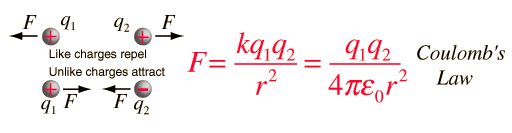# Electrostatics and Coulombines: Diagram Included.

• pugfug90

## Homework Statement

2 small spheres each having a mass of 0.1g are suspended from the same point on silk threads 20cm long. When given equal charges, they repel, resting 24cm apart. Calculate charge of each.

## Homework Equations

F(w)=mg(a)
F=kQ(1)Q(2)/d^2
k=8.987x10^9 N*m^2 / c^2

## The Attempt at a Solution

I converted grams to kilograms.. 1 x 10^-4 kg
kilograms to Newtons/weight..9.8*10^-4N

blah blah..
F=kQ(1)Q(2)/d^2
(7.35*10^-4N)(.24^2)(8.987*10^9 N*m^2/c^2)=Q^2
Q=6.86*10^-8C

http://i80.photobucket.com/albums/j199/pugfug90/physucks.gif [Broken]
Here's some work and a diagram. I am 99% sure that the answer is 6.9*10^-8 coulombs. However! I don't understand.. If I used trig to find half of the total force (in the triangle diagram).. why do I use the full distance?

Last edited by a moderator:

If I understand what you're asking: You aren't finding just half the force. Coulomb's law obeys Newton's third law, so the force exerted on Q1 by Q2 has to have the same magnitude as the force of Q2 on Q1, it is just in the opposite direction.

AHHHHHHH

But mah physical teacher drew this line between both balls (for notes) and labeled it force.. Hage, that kind of makes sense. Thanks for help. So force isn't really "half", therefore just because I'm using "half force" doesn't mean I use half distance..?

Anyone want to do the problem, starting with..

"2 small spheres each having a mass of 0.1g are suspended from the same point on silk threads 20cm long. When given equal charges, they repel, resting 24cm apart. Calculate charge of each."

Also, any online links with similar problems?
----http://i80.photobucket.com/albums/j199/pugfug90/physicismean.gif [Broken]
Because of that image, and a nice guy that kind of explained it.. I made this graphic and I hope it shows that I understand:) What do you guys think of it?

Last edited by a moderator:
Hey hey can anyone confirm my work of art?

heyheyheyheyhey